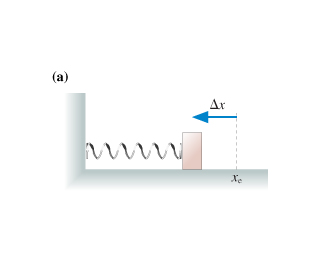# The spring in the figure (a) is compressed by length delta x . It launches the block across a frictionless surface with speed v0. The two springs in tCarol Gates 2021-02-23 AnsweredThe spring in the figure (a) is compressed by length delta x . It launches the block across a frictionless surface with speed v0. The two springs in the figure (b) are identical to the spring of the figure (a). They are compressed by the same length delta x and used to launch the same block. What is the block's speed now?
You can still ask an expert for help

• Questions are typically answered in as fast as 30 minutes

Solve your problem for the price of one coffee

• Math expert for every subject
• Pay only if we can solve itNathanael Webber
The energy stored in the spring is $0.5\cdot k\cdot \mathrm{△}{x}^{2}$ this must equal the kinetic energy of the released block.
$0.5\cdot m\cdot {v}_{0}^{2}=0.5\cdot k\cdot \mathrm{△}{x}^{2}$
${v}_{0}=\mathrm{△}x\cdot x\sqrt{\frac{2\cdot k}{m}}$
The speed from two springs is $\sqrt{2}$ times the speed with one spring.